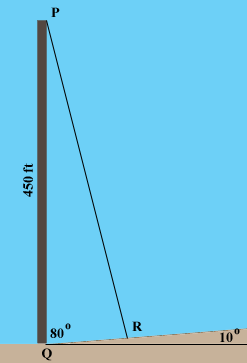SEARCH HOMEMath Central Quandaries & QueriesQuestion from Alex, a student: The height of a radio tower is 450 feet, and the ground on one side of the tower slopes upward at an angle of 10 degrees. How long should a guy wire be if it is to connect to the top of the tower and be secured at a point on the sloped side 110 feet from the base of the tower?Alex,

If the slope makes an angle of 10o with the horizontal and the tower is vertical then the tower makes an angle of 80o with the ground.In the triangle PQR you know |PQ| = 450 ft, |QR| = 110 ft and the measure of angle PQR is 80o. The Law of Cosines will then give you |RP|.

HarleyMath Central is supported by the University of Regina and The Pacific Institute for the Mathematical Sciences.